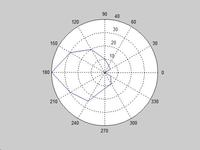# help in matlab antenna

Status
Not open for further replies.

#### cyprus

##### Banned
dipole antenna matlab

Hi dear friends im from cyprus and i find this nice web siite and i have get good informations i have a project about half wave diople antenna my friens we have data and we need to draw it in matlab and im asking for :

who can use matlab if they can help me .. how to draw polar design for half wave dipole antenna using matlab i hope someone can help me to do it i have done it by excel by now we need it by matlab as well how can we use (GUI)
example
0\0
30\5
60\6
90\10
..
..
330\5
and i would like if u can advice me by books that talks about half wave dipole antenna
i hope that i can find a help my regards to every one

draw antenna pattern in matlab

you can find this topic at any elementary antenna book.
these are some antenna book:
1- Antenna Theory: Analysis and Design - by Constantine A. Balanis
2- Antenna Theory and Design - by H. Paul Williams
3- Antenna Theory and Design - by Warren L. Stutzman, Gary A. Thiele
4- The Handbook of Antenna Design - by Alan W. Rudge
5- Antenna Engineering Handbook - by Richard C. Johnson

you can find some contents of these books by google books search

the handbook of antenna design by alan w. rudge

thanks alot i hope i will find the way how to do it in matlab thanks for ur answer ... waiting for more answers good luck

antenna +pdetool

t = 0:.01:2*pi;
polar(t,sin(2*t).*cos(2*t))

polar dipol matlab

Hi there
if you type pdetool in matlab command window, A GUI window open and you can easily draw your antenna
I hope it'll be help ful

dear pmin and ali
thanks alot for ur kind answers

assuming you have the MATLAB command interface up and running:

1. create an array of angles (in degrees) for the results:

>> a=[0 30 60 90 120 150 180 210 240 270 300 330 360]

2. create an array of pattern amplitudes (note this must have the same number of entires as the above array) - e.g.:

>> d = [0 5 6 10 20 30 40 30 25 12 10 5 0]

3. plot the results (convert degrees to radians)

polar(a*pi/180,d)

note also the following neat trick. if you already have your data recorded elsewhere (like Excel), highlight the data in (say) Excel, copy (or ctrl-C), create a variable in MATLAB as follows:

>> x=[]

place the MATLAB cursor (|) in the square brackets and paste (or ctrl-V). this will insert the data into the array without having to type the numbers.Points: 2